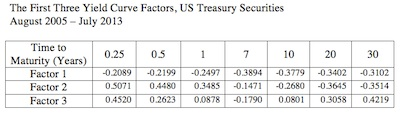### CFA Practice Question

There are 147 practice questions for this study session.

### CFA Practice QuestionWhich factor is most likely the change in the yield curve level?

A. Factor 1
B. Factor 2
C. Factor 3

It shows for a one standard deviation positive change in that factor, the yield for a 0.25-year bond would decline by 0.2089%, a 0.50-year bond by 0.2199%, and so on across maturities, so that a 30-year bond would decline by 0.3102%. It describes approximately parallel shifts up and down the entire length of the yield curve.